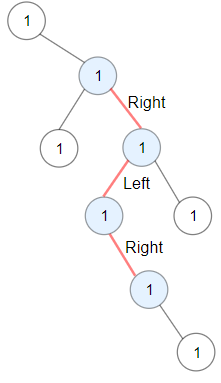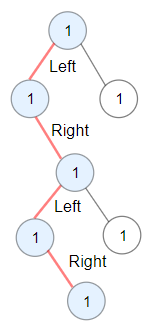## 1372. Longest ZigZag Path in a Binary Tree

Given a binary tree `root`, a ZigZag path for a binary tree is defined as follow:

• Choose any node in the binary tree and a direction (right or left).
• If the current direction is right then move to the right child of the current node otherwise move to the left child.
• Change the direction from right to left or right to left.
• Repeat the second and third step until you can't move in the tree.

Zigzag length is defined as the number of nodes visited - 1. (A single node has a length of 0).

Return the longest ZigZag path contained in that tree.

Example 1:```Input: root = [1,null,1,1,1,null,null,1,1,null,1,null,null,null,1,null,1]
Output: 3
Explanation: Longest ZigZag path in blue nodes (right -> left -> right).
```

Example 2:```Input: root = [1,1,1,null,1,null,null,1,1,null,1]
Output: 4
Explanation: Longest ZigZag path in blue nodes (left -> right -> left -> right).
```

Example 3:

```Input: root = 
Output: 0
```

Constraints:

• Each tree has at most `50000` nodes..
• Each node's value is between `[1, 100]`.

## Rust Solution

``````struct Solution;
use rustgym_util::*;

trait Postorder {
fn postorder(&self, max: &mut i32) -> (i32, i32);
}

fn postorder(&self, max: &mut i32) -> (i32, i32) {
if let Some(node) = self {
let node = node.borrow();
let (_, left_right) = node.left.postorder(max);
let (right_left, _) = node.right.postorder(max);
let left = left_right + 1;
let right = right_left + 1;
*max = (*max).max(left);
*max = (*max).max(right);
(left, right)
} else {
(0, 0)
}
}
}

impl Solution {
fn longest_zig_zag(root: TreeLink) -> i32 {
let mut res = 0;
root.postorder(&mut res);
res - 1
}
}

#[test]
fn test() {
let root = tree!(
1,
None,
tree!(
1,
tree!(1),
tree!(1, tree!(1, None, tree!(1, None, tree!(1))), tree!(1))
)
);
let res = 3;
assert_eq!(Solution::longest_zig_zag(root), res);
let root = tree!(
1,
tree!(1, None, tree!(1, tree!(1, None, tree!(1)), tree!(1))),
tree!(1)
);
let res = 4;
assert_eq!(Solution::longest_zig_zag(root), res);
let root = tree!(1);
let res = 0;
assert_eq!(Solution::longest_zig_zag(root), res);
}
``````

Having problems with this solution? Click here to submit an issue on github.